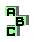ORTHOCENTROIDAL CIRCLE

 r26 Fermat points, inverse points r39 Fermat axis, Euler line r209 circumcenter, ninecenter, inverse points r210 point X(115), symmedian point, inverse points r211 Lester circle r711 ninecircle, point X(24), point X(186),inverse points r728 Exeter point, complement, point X(468), Gob point, ninecircle,inverse points r960 centroid, symmedian point, Fermat points, Apollonian circles, symmedian lines r961 orthocenter, symmedian point, Fermat points, Apollonian circles, symmedian lines r976 orthocenter r1040 incenter, symmedian point, Spieker point, Gergonne point, Nagel point, Feuerbach point, Brocard points, Fermat points, Napoleon points r1310 Johnson triangle r1521 Fermat points, isodynamic points, centroid, circumcircle r1739 circumcenter, ninecenter, perspector, point X(264), MacBeath conic, ninecircle r1821 isogonal points, tripolar, inverse points r1973 circumcenter, De Longchamps point, altitude feet, homothetic triangles, inverse points